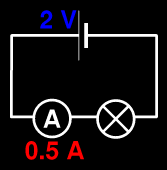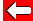# Electrical Power Example

How much energy gets to the lamp depends on how much current flows - more current means a brighter lamp. Let's look at this carefully:A voltage of 2 V means that 2 J of energy is given to every coulomb (C) of charge that passes through the cell. A current of 0.5 A means that 0.5 C of charge moves through the lamp each second. Let's look at the lamp:

The voltage across the lamp is 2 V. So for every 1 C of charge that goes into it, the lamp transfers 2 J of energy into heat and light.

But we know (by understanding current) that every second, 0.5 C of charge moves through the lamp.

Combining these two facts, we see that every second the lamp transfers 1 J of energy out of the circuit. So its power output is 1 J/s or 1 W.Go back a pageElectricity MenuGo to next page